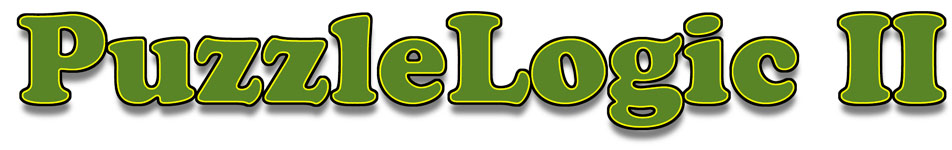Just about everybody enjoys puzzles. Unlike jig-saw puzzles, many of the puzzles in PuzzleLogic II can be solved in more than one way. Some of the puzzle can be solved in just a few moves, but figuring out which moves can be very challenging. This set of puzzles is a compilation of challenges that was specifically chosen to provide teachers, students and others to concentrate on the development of problem solving skills. Most of the solutions involve the application of arithmetic, algebraic, geometric and logical skills.

Keys to Problem Solving

Most of the puzzles can be solved in more than one way. A strategy that is appropriate for one type of problem may not work for other problems. Here are some examples of the different problem solving strategies that will be helpful.

• Look for a pattern.
• Guess an answer and check result.
• Write an expression or equation.
• Use logical reasoning.
• Work the problem backwards.
• Make a diagram or drawing.
• Make a list, sort it and organize it.
• Make a table or spreadsheet.
• Use a tree diagram.
• Solve a simpler problem which is related.
• Imagine that you are a component of the problem and act it out.
• Sleep on it.

PuzzleLogic II consists of twelve different puzzles. The puzzles are solved by moving markers on the screen until a solution is found.

Thinking through the puzzle and developing a strategy or plan of attack will assist in finding a solution. Sometimes the most difficult part of the puzzle involves visualizing the pattern which is at the core of the puzzle. Some puzzles are similar to others so the ability to find a solution will improve through practice.

Puzzles:

1. Cross Sums
2. Elegant Octagon
3. Magic Square
4. Number Star
5. Triangle Sums
6. Honeycomb
7. Lucky Stars
8. Sum Fun
9. Around We Go!
10. Money Magic
11. Computo
12. Circle of Sums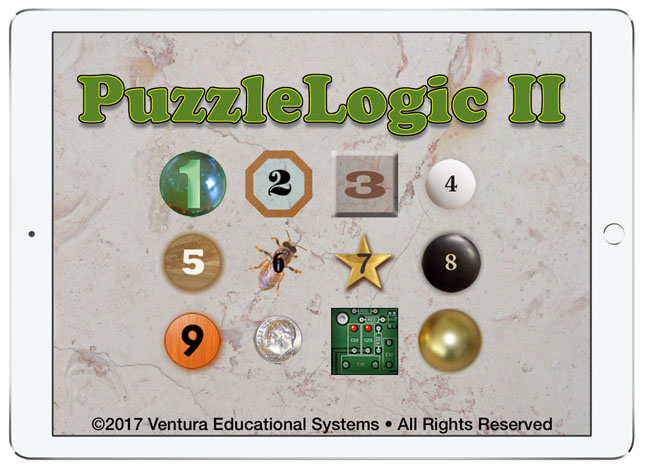Features: Simulated wood and tile puzzle boards an puzzle pieces Sound effects and speech A counter reports the number of moves used to solve a puzzle On/Off options for select features \$1.99 "I have included PuzzleLogic in our recommended iPad apps list. I find it a very innovative app that will keep students engaged." Lori Gracey, TCEA.org (Texas Computers in Education Association)
Sample Puzzles

Cross Sums

Cross Sums puzzles are a great way to focus on problem solving strategies.The object of this puzzle is to arrange numbers along the horizontal and vertical lines of the puzzle to make the sums along each line the same. This puzzle can be solved in more than one way. How many ways can you find?

Money Magic

Arrange the quarters, dimes and nickels along the four lines of this puzzle so that the total values of the coins along each line are the same.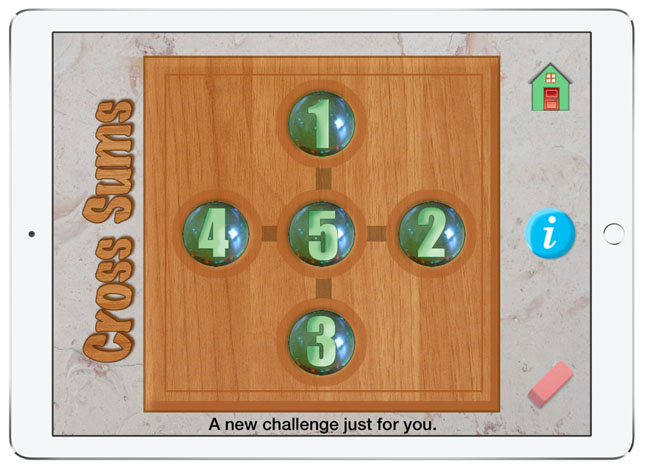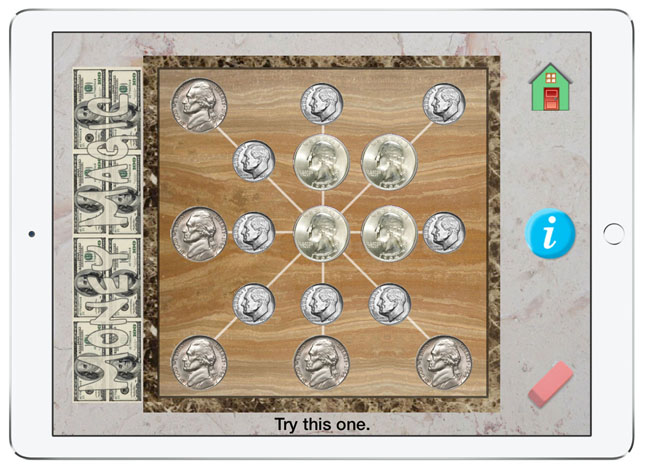Computo

Using just 1, 2, 3 & 4, and the basic operations, add, subtract, multiply and divide, can you make all the numbers from 1 to 10? Change the operation by tapping the gray up and down arrows. Drag and drop to rearrange the numbers.

Magic Square

Magic Squares are fascinating math puzzles. The object of this puzzle is to arrange the numbers from 1 to 9 along the vertical and horizontal lines so that each row and column have the same sum. For an extra challenge include the two diagonals.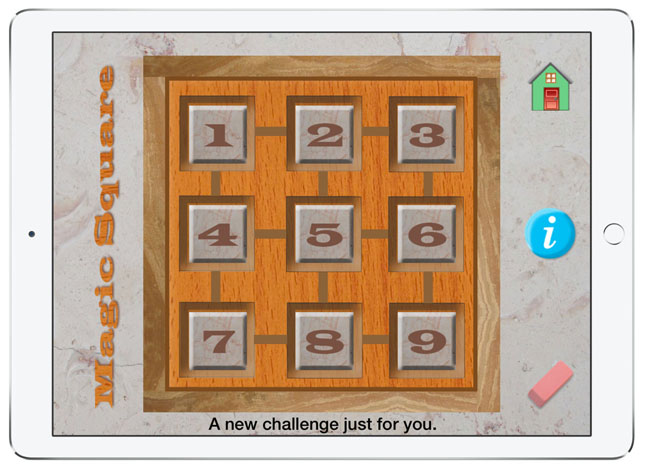PuzzleLogic II is now available at the Apple iTunes Store.